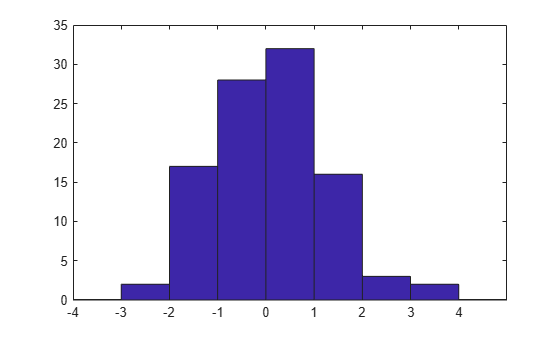# histc

Histogram bin counts (not recommended; use `histcounts`)

`histc` is not recommended. Use `histcounts` instead.

For more information, including suggestions on updating code, see Replace Discouraged Instances of hist and histc.

## Syntax

``bincounts = histc(x,binranges)``
``bincounts = histc(x,binranges,dim)``
``````[bincounts,ind]= histc(___)``````

## Description

example

````bincounts = histc(x,binranges)` counts the number of values in `x` that are within each specified bin range. The input, `binranges`, determines the endpoints for each bin. The output, `bincounts`, contains the number of elements from `x` in each bin. If `x` is a vector, then `histc` returns `bincounts` as a vector of histogram bin counts.If `x` is a matrix, then `histc` operates along each column of `x` and returns `bincounts` as a matrix of histogram bin counts for each column. To plot the histogram, use `bar(binranges,bincounts,'histc')`. ```
````bincounts = histc(x,binranges,dim)` operates along the dimension `dim`.```

example

``````[bincounts,ind]= histc(___)``` returns `ind`, an array the same size as `x` indicating the bin number that each entry in `x` sorts into. Use this syntax with any of the previous input argument combinations.```

## Examples

collapse all

Initialize the random number generator to make the output of `randn` repeatable.

`rng(0,'twister')`

Define `x` as 100 normally distributed random numbers. Define bin ranges between -4 and 4. Determine the number of values in `x` that are within each specified bin range. Return the number of elements in each bin in `bincounts`.

```x = randn(100,1); binranges = -4:4; [bincounts] = histc(x,binranges)```
```bincounts = 9×1 0 2 17 28 32 16 3 2 0 ```

To plot the histogram, use the `bar` function.

```figure bar(binranges,bincounts,'histc')```Defined `ages` as a vector of ages. Sort `ages` into bins with varying ranges between 0 and 75.

```ages = [3,12,24,15,5,74,23,54,31,23,64,75]; binranges = [0,10,25,50,75]; [bincounts,ind] = histc(ages,binranges)```
```bincounts = 1×5 2 5 1 3 1 ```
```ind = 1×12 1 2 2 2 1 4 2 4 3 2 4 5 ```

`bincounts` contains the number of values in each bin. `ind` indicates the bin numbers.

## Input Arguments

collapse all

Values to be sorted, specified as a vector or a matrix. The bin counts do not include values in `x` that are `NaN` or that lie outside the specified bin ranges. If `x` contains complex values, then `histc` ignores the imaginary parts and uses only the real parts.

Data Types: `single` | `double` | `int8` | `int16` | `int32` | `uint8` | `uint16` | `uint32`

Bin ranges, specified as a vector of monotonically nondecreasing values or a matrix of monotonically nondecreasing values running down each successive column. The values in `binranges` determine the left and right endpoints for each bin. If `binranges` contains complex values, then `histc` ignores the imaginary parts and uses only the real parts.

If `binranges` is a matrix, then `histc` determines the bin ranges by using values running down successive columns. Each bin includes the left endpoint, but does not include the right endpoint. The last bin consists of the scalar value equal to last value in `binranges`.

For example, if `binranges` equals the vector `[0,5,10,13]`, then `histc` creates four bins. The first bin includes values greater than or equal to 0 and strictly less than 5. The second bin includes values greater than or equal to 5 and less than 10, and so on. The last bin contains the scalar value 13.

Data Types: `single` | `double` | `int8` | `int16` | `int32` | `uint8` | `uint16` | `uint32`

Dimension along which to operate, specified as a scalar.

## Output Arguments

collapse all

Number of elements in each bin, returned as a vector or a matrix. The last entry in `bincounts` is the number of values in `x` that equal the last entry in `binranges`.

Bin index numbers, returned as a vector or a matrix that is the same size as `x`.

## Tips

• If values in `x` lie outside the specified bin ranges, then `histc` does not include these values in the bin counts. Start and end the `binranges` vector with `-inf` and `inf` to ensure that all values in `x` are included in the bin counts.

## Version History

Introduced before R2006a